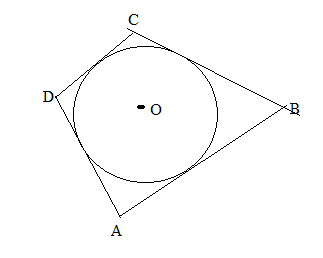Chapter 6.3, Problem 29EElementary Geometry For College St...

7th Edition
Alexander + 2 others
ISBN: 9781337614085

Solutions

Chapter
SectionElementary Geometry For College St...

7th Edition
Alexander + 2 others
ISBN: 9781337614085
Textbook Problem

In Exercises 27 to 30, provide a paragraph proof.Given: Quadrilateral ABCD is circumscribed about ⊙ O Prove: A B + C D = D A + B C .To determine

To find:

To prove AB+CD=DA+BC.

Explanation

Given that, Quadrilateral ABCD is circumscribed about O.

To prove AB+CD=DA+BC

The diagrammatic representation is given below,

From the above the circle shows that tangents to a circle from the same point are equidistant.

The diagrammatic representation is given below,

We know that,

AB+CD=x+y+z+w..

Still sussing out bartleby?

Check out a sample textbook solution.

See a sample solution

The Solution to Your Study Problems

Bartleby provides explanations to thousands of textbook problems written by our experts, many with advanced degrees!

Get Started

Prove the statement using the precise definition of a limit. limx4+2x4=

Single Variable Calculus: Early Transcendentals, Volume I

For y=12gt2 (where g is a constant), dydt=. a) 14g2 b) 12g c) gt d) gt2

Study Guide for Stewart's Single Variable Calculus: Early Transcendentals, 8th

In Exercises 14, write the set in set-builder notation. {3,4,5,6,7}

Finite Mathematics for the Managerial, Life, and Social Sciences